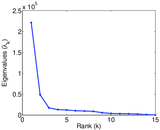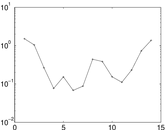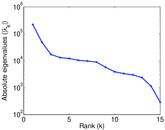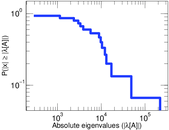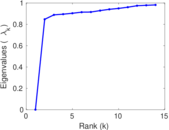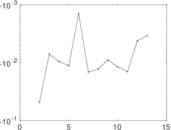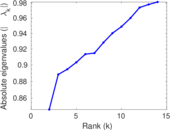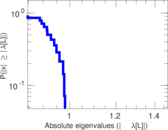# Discogs artist–genre

Discogs (short for "discographies") is a large online music database that provides information about audio records including information about artists, labels and release details. Each edge of this bipartite network connects an artist and a genre. An edge indicates that the artist was involved in the production of a release of this genre. The left nodes represent artists and the right nodes represent genres.

 Code `Da` Internal name `discogs_genre` Name Discogs artist–genre Data source http://www.discogs.com/ AvailabilityDataset is available for download Consistency checkDataset passed all tests Category Feature network Node meaning Artist, genre Edge meaning Feature Network formatBipartite, undirected Edge typeUnweighted, multiple edges

## Statistics

 Size n = 1,754,838 Left size n1 = 1,754,823 Right size n2 = 15 Volume m = 19,033,891 Unique edge count m̿ = 3,142,059 Wedge count s = 713,800,298,659 Claw count z = 151,287,735,212,950,336 Cross count x = 2.700 85 × 1022 Square count q = 111,241,556,929 4-Tour count T4 = 3,745,144,849,158 Maximum degree dmax = 5,837,587 Maximum left degree d1max = 392,118 Maximum right degree d2max = 5,837,587 Average degree d = 21.693 0 Average left degree d1 = 10.846 6 Average right degree d2 = 1.268 93 × 106 Fill p = 0.119 369 Average edge multiplicity m̃ = 6.057 78 Size of LCC N = 1,754,837 Diameter δ = 4 50-Percentile effective diameter δ0.5 = 3.186 26 90-Percentile effective diameter δ0.9 = 3.837 25 Median distance δM = 4 Mean distance δm = 3.228 90 Gini coefficient G = 0.892 468 Balanced inequality ratio P = 0.118 928 Left balanced inequality ratio P1 = 0.182 595 Right balanced inequality ratio P2 = 0.258 492 Relative edge distribution entropy Her = 0.618 216 Power law exponent γ = 3.536 90 Tail power law exponent γt = 3.361 00 Tail power law exponent with p γ3 = 3.361 00 p-value p = 0.000 00 Left tail power law exponent with p γ3,1 = 3.361 00 Left p-value p1 = 0.000 00 Right tail power law exponent with p γ3,2 = 6.771 00 Right p-value p2 = 0.000 00 Degree assortativity ρ = −0.284 127 Degree assortativity p-value pρ = 0.000 00 Spectral norm α = 221,106 Algebraic connectivity a = 0.846 666 Spectral separation |λ1[A] / λ2[A]| = 4.534 39 Controllability C = 1,754,808 Relative controllability Cr = 0.999 983

## Plots

### Fruchterman–Reingold graph drawing### Degree distribution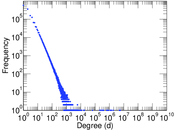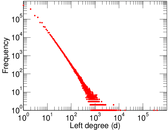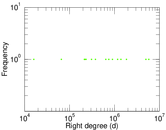### Cumulative degree distribution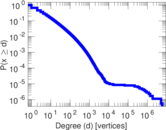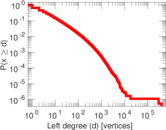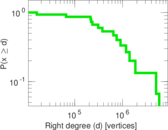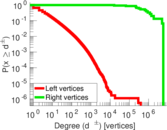### Lorenz curve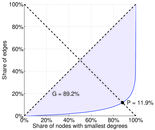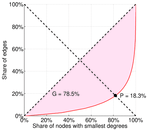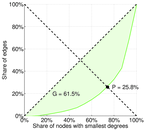### Spectral distribution of the adjacency matrix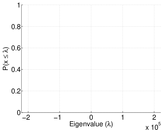### Spectral distribution of the normalized adjacency matrix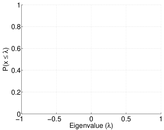### Spectral distribution of the Laplacian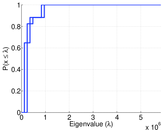### Spectral graph drawing based on the adjacency matrix### Spectral graph drawing based on the Laplacian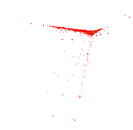### Spectral graph drawing based on the normalized adjacency matrix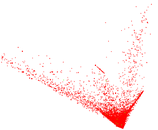### Degree assortativity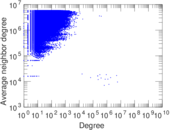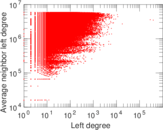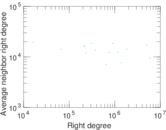### Zipf plot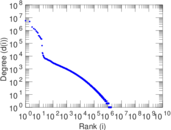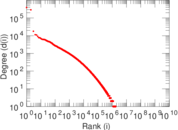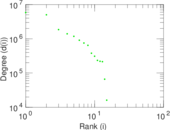### Hop distribution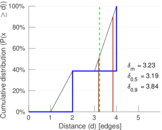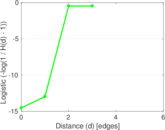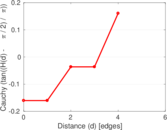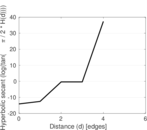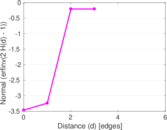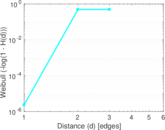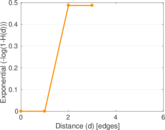### Delaunay graph drawing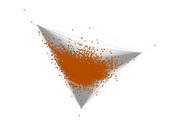### Edge weight/multiplicity distribution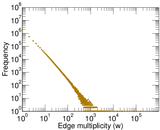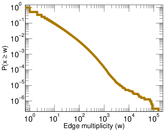### Matrix decompositions plots